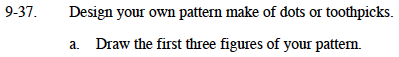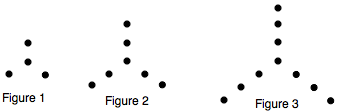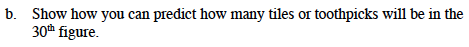### Home > MC1 > Chapter 9 > Lesson 9.1.3 > Problem9-37

9-37.Everyone's dots will look different, but make sure there isa pattern. Sharon's first three figures are below.For each figure of Sharon's pattern, there are 1+ 3x dots, where x is the figure number.
In the 30th Figure, there are 91 dots, since 1+3(30) = 91.
Try writing a similar expression for the number of dots in your pattern.